# Term Frequencies and TF-IDF: How are these calculated?

12Administrator, Moderator, Employee, RapidMiner Certified Analyst, Community Manager, Member, University Professor, PM Moderator Posts: 2,717Community Manager
edited March 2019

## Term Frequencies and TF-IDF: How are these calculated?

Are you are like me – using "Process Documents" for a long time but never truly understood what those numbers are? Or perhaps just a math geek who enjoys vector analysis? Either way, relax for a moment while I walk you through a step-by-step example of exactly how Term Occurrences, Term Frequencies (TF), and Term Frequency-Inverse Document Frequencies (TF-IDF) work in RapidMiner...

## Set Up Example

We're going to start with a VERY simple example: two short documents in one corpus. Document 1 says "This is a report about data mining and text mining" and Document 2 says "This is a text about video analysis".  I am going to combine these two documents and run them through "Process Documents" with Tokenization, Transform Cases, and Filter Stopwords (English):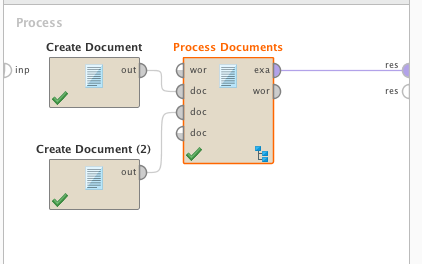Main process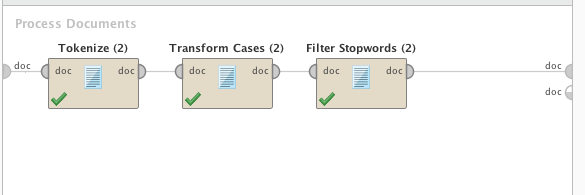Inside "Process Documents"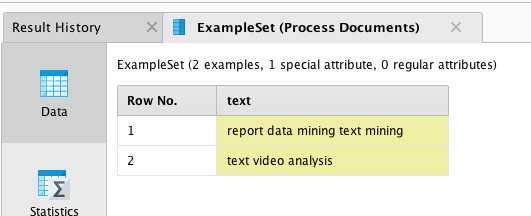Results without word vectors

## Term Occurrences

This is the most basic word token vectorization you can do. It simply counts the word token occurrences in each document to create a "term occurrence word vector":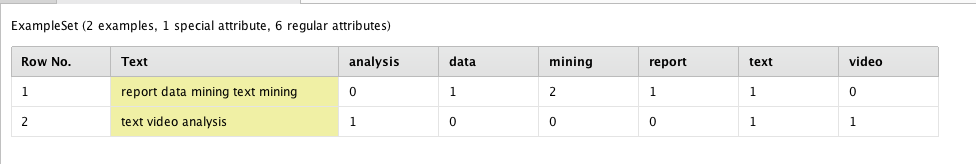Term Occurrences for sample corpus of 2 documents

## Binary Term Occurrences

This is also rather straightforward. The only difference is that the only options are 0 and 1. Hence you will see that the only change is in Document 1, RapidMiner changes the "2" to a "1", simply indicating that there is at least one occurrence of the word token "mining".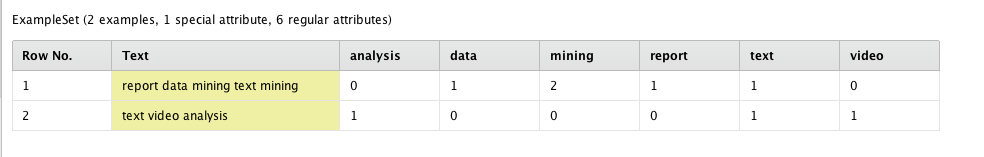Binary Term Occurrences for sample corpus of 2 documents

## Term Frequencies

This is where things get interesting. At the most basic level, Term Frequency (TF) is simply the ratio of the occurrence of each word token to the total number of word tokens in the document. First I use the Extract Token Number operator inside the Process Documents to get the total number of word tokens in each document: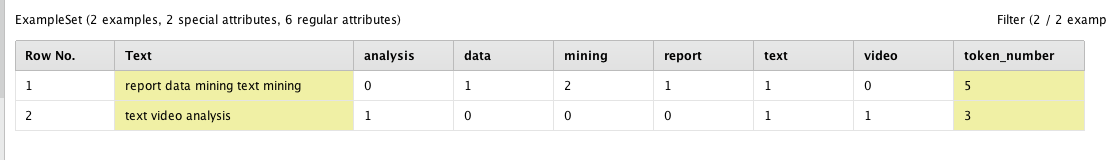Results from using the "Extract Token Number" in the Process Documents operator to get the token count for each document in the corpus

Now Term Frequencies should make sense: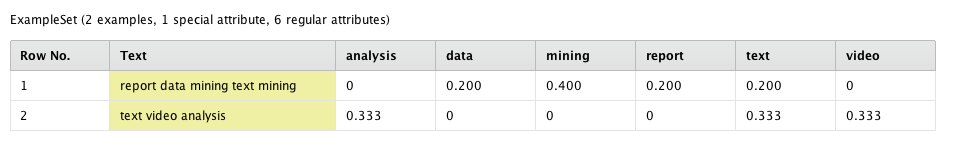Term Frequencies for sample corpus of 2 documents

But you may notice that this is NOT what RapidMiner gives you when you select "Term Frequencies" in the "Vector Creation" parameter: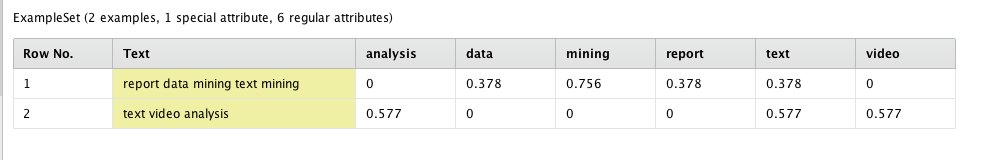RapidMiner result for Term Frequencies with sample corpus of 2 documents

Why? Because the term frequency word vectors that are shown in RapidMiner are normalized vectors. This is exactly the same as unit vector normalization that you may have seen in physics classes.  In broad brush strokes, the norm of a (Euclidean) vector is its length or size. If you have a 1x2 vector, you can find the norm by simple Pythagorean Theorem. For a 1x6 vector like each document above, you use Pythagorean Theorem but in 6-dimensional space.  Hence the norm of the first document term frequency vector is:

`SQRT [ (0)^2 + (0.200)^2 + (0.400)^2 + (0.200)^2 + (0.200)^2 + (0)^2 ] = 0.529`

and the second document term frequency vector is:

`SQRT [ (0.333)^2 + (0)^2 + (0)^2 + (0)^2 + (0.333)^2 + (0.333)^2 ] = 0.577`

In order to look at all the documents equally, we want all the document vectors to have the same length. So we divide each document term frequency vector by its respective norm to get a "document term frequency unit vector" – also called a normalized term frequency vector.

So if we divide each term frequency in each document term frequency vector by its norm:

`0 / 0.529 = 0           0.200 / 0.529 = 0.378     0.400 / 0.529 = 0.756     0.200 / 0.529 = 0.378 ....0.333 / 0.577 = 0.577   0 / 0.577 = 0             0 / 0.577 = 0             0 / 0.577 = 0 ....`

we will get normalized term frequency vectors for each document – exactly what RapidMiner shows.RapidMiner result for Term Frequencies with sample corpus of 2 documents

## Inverse Document Frequency (IDF)

OK but why go beyond TFs at all? Well the problem with TFs is that they only look at word tokens relative to other word tokens in a single document, not relative to the entire corpus. And usually in text mining you want to train your ML model on a large number of documents, not one.  Hence finding word tokens that are particularly strong among many documents is far more important than each document in isolation.

But what is strong? It is exactly like weighting attributes in normal machine learning; word tokens that are able to distinguish one document from another should be weighted heavier than others that do not provide this insight, i.e. we weight a word token's uniqueness rather than its popularity.

Say you had a library of books and wished to train a model that classified books based on "has animals" vs "has no animals".  Clearly word tokens like "tiger", "elephant" and "bear" are far more important than word tokens like "if", "or" and "because".  So we call this unique strength factor an inverse document frequency: the less frequent it occurs, the stronger it should be.

So let's look at our corpus. We have two documents with a total of six unique word tokens: analysis, data, mining, report, text, and video. Note that the word token "text" occurs in both documents – its predictive strength should not be as large as the other word tokens which are unique to each document.  We could do something as simple as this:

`analysis: 1 out of 2 documents = 1/2data: 1 out of 2 documents = 1/2mining: 1 out of 2 documents = 1/2report: 1 out of 2 documents = 1/2text: 2 out of 2 documents = 2/2video: 1 out of 2 documents = 1/2`

but we want "text" to be stronger, so let's use exponents:

`analysis: 1 out of 2 documents = e^(1/2) = 1.649data: 1 out of 2 documents = e^(1/2) = 1.649mining: 1 out of 2 documents = e^(1/2) = 1.649report: 1 out of 2 documents = e^(1/2) = 1.649text: 2 out of 2 documents = e^(2/2) = 2.718video: 1 out of 2 documents = e^(1/2) = 1.649`

but that's not a good idea. Imagine if you had 100 documents and only one had "video"; the others are in 99 out of the 100 documents and hence far less important; you would want "video" to be very heavily weighted against them.  This does not work well at all.

`analysis: 99 out of 100 documents = e^(99 / 100) = 2.691data: 99 out of 100 documents = e^(99 / 100) = 2.691mining: 99 out of 100 documents = e^(99 / 100) = 2.691report: 99 out of 100 documents = e^(99 / 100) = 2.691text: 99 out of 100 documents = e^(99 / 100) = 2.691video: 1 out of 100 documents = e^(1 / 100) = 1.01`

However if you flip it around and find ln of the reciprocal, it works like you would expect:

`analysis: 99 out of 100 documents = ln (100 / 99) = ln 1.01 = 0.01data: 99 out of 100 documents = ln (100 / 99) = ln 1.01 = 0.01mining: 99 out of 100 documents = ln (100 / 99) = ln 1.01 = 0.01report: 99 out of 100 documents = ln (100 / 99) = ln 1.01 = 0.01text: 99 out of 100 documents = ln (100 / 99) = ln 1.01 = 0.01video: 1 out of 100 documents = ln (100 / 1) = ln 100 = 4.605`

here the word token "video" is weighted approximately 461 times heavier than the other word tokens. That makes sense.

Now let's look at our original example with our one IDF vector. As you can see, the word token "text" has much less strength than the others who all have equal strength (or weight), as it should be.

`analysis: 1 out of 2 documents = ln (2 / 1) = 0.693data: 1 out of 2 documents = ln (2 / 1) = 0.693mining: 1 out of 2 documents = ln (2 / 1) = 0.693report: 1 out of 2 documents = ln (2 / 1) = 0.693text: 2 out of 2 documents = ln (2 / 2) = 0video: 1 out of 2 documents = ln (2 / 1) = 0.693∴IDF vector = (0.693   0.693   0.693   0.693   0   0.693)`

## Term Frequency – Inverse Document Frequency (TF-IDF)

So now let's put it all together by multiplying each term frequency vectors by the inverse document frequency vector (represented as a diagonal matrix):

`Document 1 TF-IDF:(0   0.2   0.4   0.2   0.2   0)    x    (0.693      0        0        0        0       0  )                                        (  0      0.693      0        0        0       0  )                                        (  0        0      0.693      0        0       0  )                                        (  0        0        0        0        0       0  )                                                                                         (  0        0        0        0      0.693     0  )                                        (  0        0        0        0        0     0.693)= (0   0.138   0.277   0.138   0   0)Document 2 TF-IDF:(0.333   0   0   0   0.333   0.333) x   (0.693      0        0        0        0       0  )                                        (  0      0.693      0        0        0       0  )                                        (  0        0      0.693      0        0       0  )                                        (  0        0        0        0        0       0  )                                                                                         (  0        0        0        0      0.693     0  )                                        (  0        0        0        0        0     0.693)= (0.231    0    0    0    0    0.231)`

And now all that is left is to normalize these TF-IDF vectors like we did for TF:

`SQRT [ (0)^2 + (0.138)^2 + (0.277)^2 + (0.138)^2 + (0)^2 + (0)^2 ] = 0.340SQRT [ (0.231)^2 + (0)^2 + (0)^2 + (0)^2 + (0)^2 + (0.231)^2 ] = 0.327`
`Document 1: 0 / 0.340 = 0           0.138 / 0.340 = 0.408     0.277 / 0.340 = 0.816     0.138 / 0.340 = 0.408 ....Document 2: 0.231 / 0.327 = 0.707   0 / 0.327 = 0             0 / 0.327 = 0             0 / 0.327 = 0 ....`

Which is exactly what RapidMiner gives us for TF-IDF!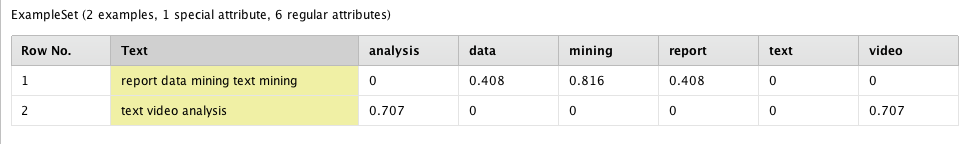RapidMiner result for TF-IDF with sample corpus of 2 documents

I hope you enjoyed that as much as I did.Scott

• Member Posts: 1Contributor I

Very very informative, Scott. I get it. Thanks.

Suppose I have a corpus of 2500 documents (essentially a text column in a data set) and I do td-idf analysis on it and get the inverse frequecy vector for all the terms in all the rows (texts).

Then I add a new text row and want to "score" this row against the other 2500 as to how similar it is to each of them. How do I set up RM to do this analysis? Is there a way for td-idf to do the analysi and get a score so can see how "similar" my test row is top teach of the other "texts" (or rows)?

Andres Fortino

• 12Administrator, Moderator, Employee, RapidMiner Certified Analyst, Community Manager, Member, University Professor, PM Moderator Posts: 2,717Community Manager

hi Andres -

Oh you want to see similarity to EACH row? I'd just use the Data to Similarity operator...• Member Posts: 33Contributor II

you written " you would want "video" to be very heavily weighted against them", So that means I need a label for weighting, so he can work with it?
br
• 12Administrator, Moderator, Employee, RapidMiner Certified Analyst, Community Manager, Member, University Professor, PM Moderator Posts: 2,717Community Manager
hi @Flixport no - the scalar value of "video" in each vector is the weight itself. It does not need a label.